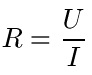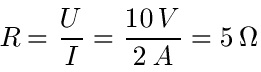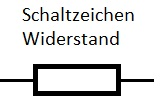# The resistance depends on the temperature

## Electrical resistance: formula, definition etc.

Electrical resistance can be viewed from many angles. This includes a definition and a formula for calculating the resistance. Further topics are the reciprocal value (Siemens), the temperature dependence of resistors, changeable resistors and their circuit symbols, the conductivity and the resistance of conductors. This article belongs to our field of physics or electrical engineering.What is actually meant by electrical resistance? Let's start with a definition: electrical resistance is a measure of the electrical voltage required to allow a certain electrical current to flow through an electrical conductor (resistance). Whereby you have to be a little careful with the term resistor: There is also a component which is called a resistor and which can be found in almost every electrical circuit.

The causes of the electrical resistance are, for example, irregular heat oscillations of the atoms as well as disturbances in the structure of the crystal lattice. How great the resistance is depends, for example, on the material used, its structure and the temperature. An R is usually used as a symbol for the electrical resistance. The unit is ohm, the unit symbol is a large omega.

Show:

### Ohm's law and symbols

Let's look at the most important law in electrical engineering: Ohm's law. You should be aware of the following: An electrical resistance is an ohmic resistance if its value is independent of the strength of the flowing current, the electrical voltage and the frequency. The equation for calculating the resistance is then:It applies:

• "R" is the resistance in ohms
• "U" is the voltage in volts
• "I" is the current in amps

Hints:

• If you are not familiar with electricity, please see the article on electricity.

example 1:

The voltage is 10 volts, the current is 2 amps. How great is the resistance?How do you draw an electrical resistance in a circuit? Well, the circuit symbol looks like this:Show:

### Electrical resistance topics

There are a large number of topics related to electrical resistance. Here you will find a short introduction to other topics related to electrical resistance and - if the article is already available - a link to detailed information.

• Ohm's law: We had a brief introduction to Ohm's law here. You can find more on this topic in the article Ohm's Law.
• Specific resistance: The specific resistance is a temperature-dependent material constant with the symbol ρ (Rho). It indicates the resistance of an electrical conductor made of a material that is 1 m long and has a continuous cross-sectional area of ​​1 mm2 having. More on this in the article specific resistance.
• Electrical conductance: In addition to electrical resistance, there is also electrical conductance (in Siemens). This is understood to be the reciprocal of the resistance. You can find the article under electrical conductance.
• conductivity: Electrical conductivity, also known as conductivity, is a physical quantity that indicates the ability of a substance to conduct electricity. See also the article electrical conductivity.
• Temperature-dependent resistance: Resistances are temperature dependent. How to calculate the change in resistance when the temperature changes is a separate chapter. More on this in the article temperature resistances / temperature dependency.
• Changeable resistances: There are resistors that are adjustable in size. Something like that is called a potentiometer. I am currently working on an article on the subject. As soon as it is available it will be linked here.
• AC resistance: The impedance, also known as alternating current resistance, indicates the relationship between the electrical voltage at a consumer and the current drawn. I am currently working on an article on the subject. As soon as it is available it will be linked here.

Left:

### Who's Online

We have 1920 guests online